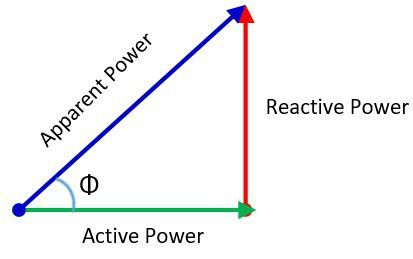# Difference Between Active & Reactive Power

The most significant difference between the active and reactive power is that the active power is the actual power which is dissipated in the circuit. Whereas, the reactive power is the useless power which only flows between the source and load. The other differences between the active and reactive power are explained below in the comparison chart.

The active, apparent and real power induces in the circuit only when their current lags behind the applied voltage by an angle of Φ. The right-angled triangle shown below shows the relation between the active, reactive and apparent power.Where, S – apparent power
Q – reactive power
P – Active power

## Comparison Chart

Basis for Comparison Active Power Reactive Power
Definition The active power is the real power which is dissipated in the circuit. The power which moves back and froth between the load and source such type of power is known as the reactive power
FormulaMeasuring Unit WattsVAR
Represented By PQ
Causes Produces heat in heater, light in lamps and torque in motor.Measures the power factor of the circuit.
Measuring Instrument Wattmeter VAR Meter

### Definition of Active Power

The power which is dissipated or do the useful work in the circuit is known as the active power. It is measured in watts or megawatts. The active power is denoted by the capital alphabet P. The average value of power in the circuit is given by the expression.The active power derives the circuit and load.

### Definition of Reactive Power

The reactive power moves between the source and load of the circuit. This power is not doing any useful works on the load. Q represents the reactive power, and it is measured in VAR. The reactive power is stored in the circuit, and it is discharged by the induction motor, transformer or by solenoids.

### Key Differences between Active and Reactive Power

1. The active power is the real power consumes by the load. Whereas, the reactive power is the useless power.
2. The active power is the product of the voltage, current and the cosine of the angle between them. Whereas, the reactive power is the product of voltage and current and the sine of the angle between them.
3. The active power is the real power, and it is measured in watts. While the reactive power is measured in VAR.
4. The letter P represents the Active power, and the Q represents the reactive power.
5. The torque that develops in the motor, the heat dissipated in the heater and the light that emit through the lamps all these produces because of the active power. The reactive power determines the power factor of the circuit.
6. The wattmeter measures the active power, and the VAR meter is used for measuring the apparent power.

### Conclusion

The active power does the useful work in the circuit. And the reactive power merely flows in the circuit without doing any useful work.